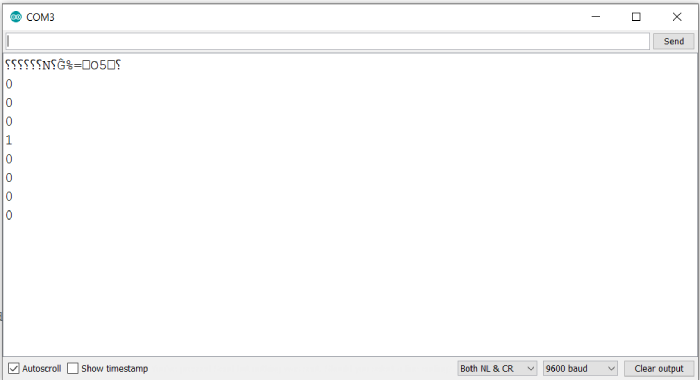# Read a specific bit of a number with Arduino

Each number has a specific binary representation. For example, 8 can be represented as 0b1000, 15 can be represented as 0b1111, and so on. If you wish to read a specific bit of a number, Arduino has an inbuilt method for it.

## Syntax

bitRead(x, index)

where, x is the number whose bits you are reading, index is the bit to read. 0 corresponds to least significant (right-most) bit, and so on.

This function returns either 0 or 1 depending on the value of that bit in that number.

## Example

The following example will illustrate the use of this function −

void setup() {
// put your setup code here, to run once:
Serial.begin(9600);
Serial.println();
int x = 8;

}

void loop() {
// put your main code here, to run repeatedly:
}

## Output

The Serial Monitor output is shown below −As you can see, only the bit in position 3 is 1, while all others are 0, which corresponds to the binary representation of 8: 0b00001000